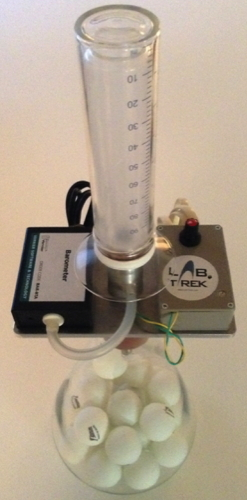# Experiment of Rüchardt for measurement of γ = cp/cv

 Rüchardt Experiment for measuring γ=cp/cv The purpose of this experience is the measure of value for γ = cp/cv in air, using a modern version of the Rüchardt method (1929). This method exploits the properties of adiabatic transformations of an ideal gas following the PVγ = const equation where the coefficient γ is equal to the ratio cp/cv In the original version of the experiment were carried out a series of compressions and expansions reversible adiabatic air mass, by swinging a ball into a thin glass rod connected to a flask, and measuring only the frequency of oscillation of the sphere. The use of the technique allows to enrich the RTL experiment allowing an inspection of the nature of the transformations carried out in gas, by monitoring the progress of all three thermodynamic variables P, V, T. The apparatus consists of a flask is connected to an external oscillating piston of section A (Figure 1). Volume trends over time is measured using a Sonar located above the oscillating piston, while a pressure sensor is connected to the volume of air in the flask.Experiments:Study of an adiabatic transformation Measurement of the ratio cp/cv for air For measuring the temperature, (not being usable the commercial sensors given the needed speed of response ), an ad hoc sensor was created consisting of a tungsten wire coupled to a special signal conditioning circuitry. In Figure 2 shows an example of trends over time in pressure and temperature of the gas piston displacement (volume) obtained with 3 sensors. For small changes in pressure dP and dV the Poisson relation in differential form is dP =-(γP/V) dV By moving the piston of x amount from the equilibrium position, the corresponding change in volume is dV = xA and the corresponding force acting on the piston is F = AdP =-A2 x γ P/V. The oscillations of the piston are then almost harmonics. A graph of dP/P0 versus dV/V0, is a straight line with slope -γ (Figure 3). The nature of transformation from the gas can be checked by comparing trends over time of relative variations of the PV product (constant for an isotherm) and PVγ (constant for an adiabatic). The dynamics can be modulated by changing the oscillating weight m (loading the piston with metal rings) or the effective air volume V (introducing ping-pong balls in the flask).Figure 1Figure 2Figure 3 Items supplied: Flask Piston Temperature sensor Position sensor Pressure sensor Metallic rings Ping-pongMaterial required but not provided: PC , DataLogger and Software for data collection

see: A new MBLversion of the Rüchardt experiment,  Am. J. Phys. 69, (2001)